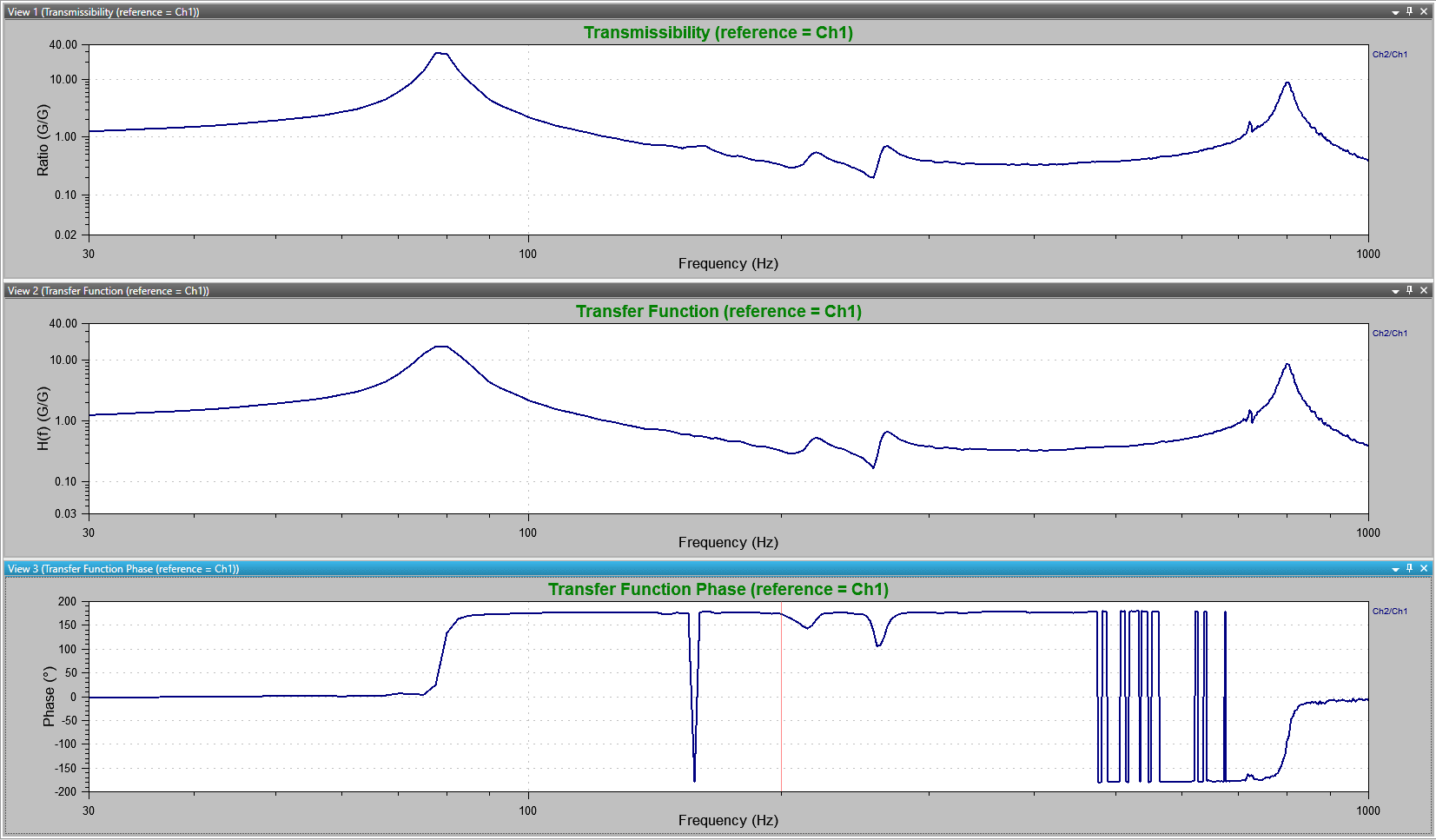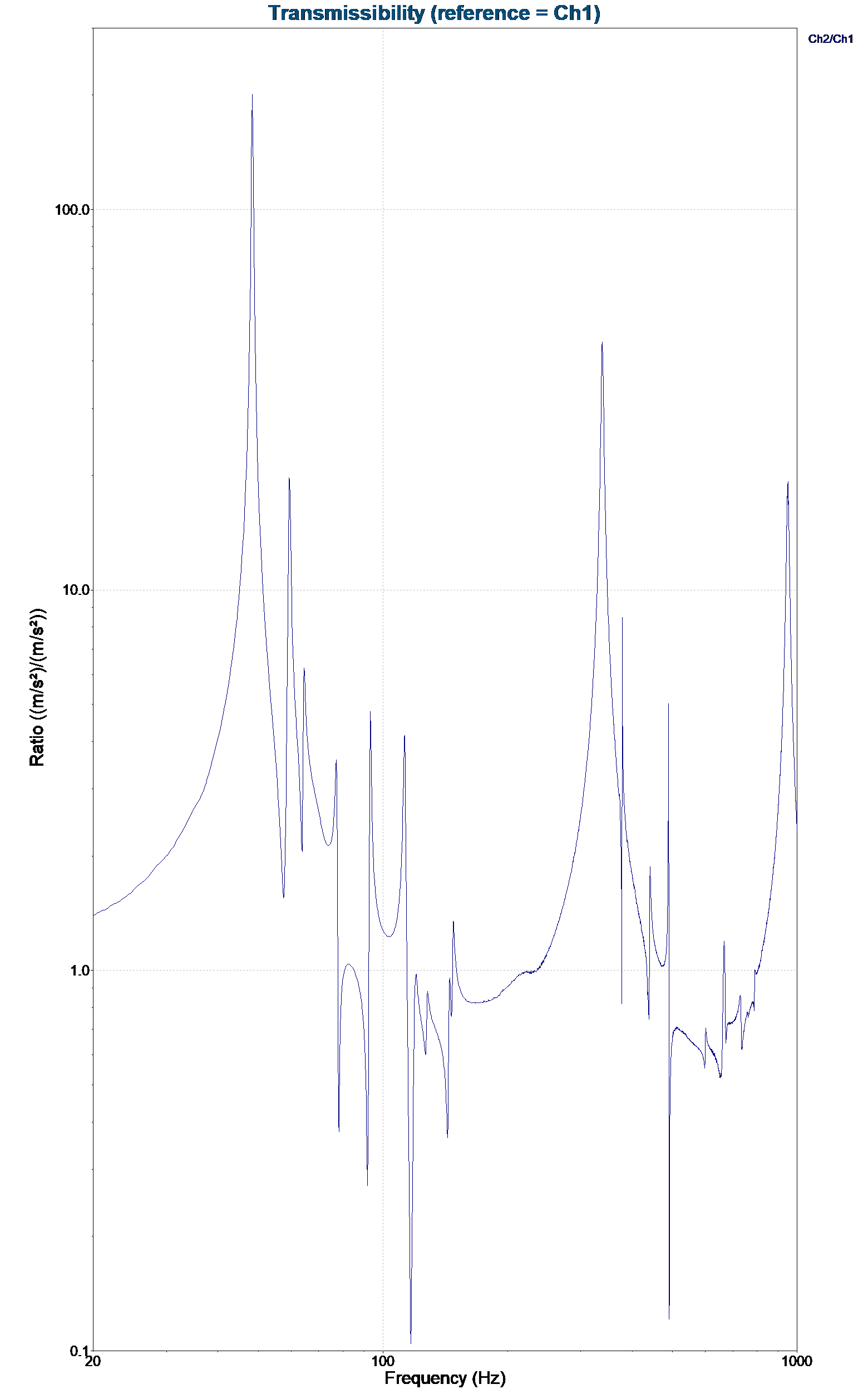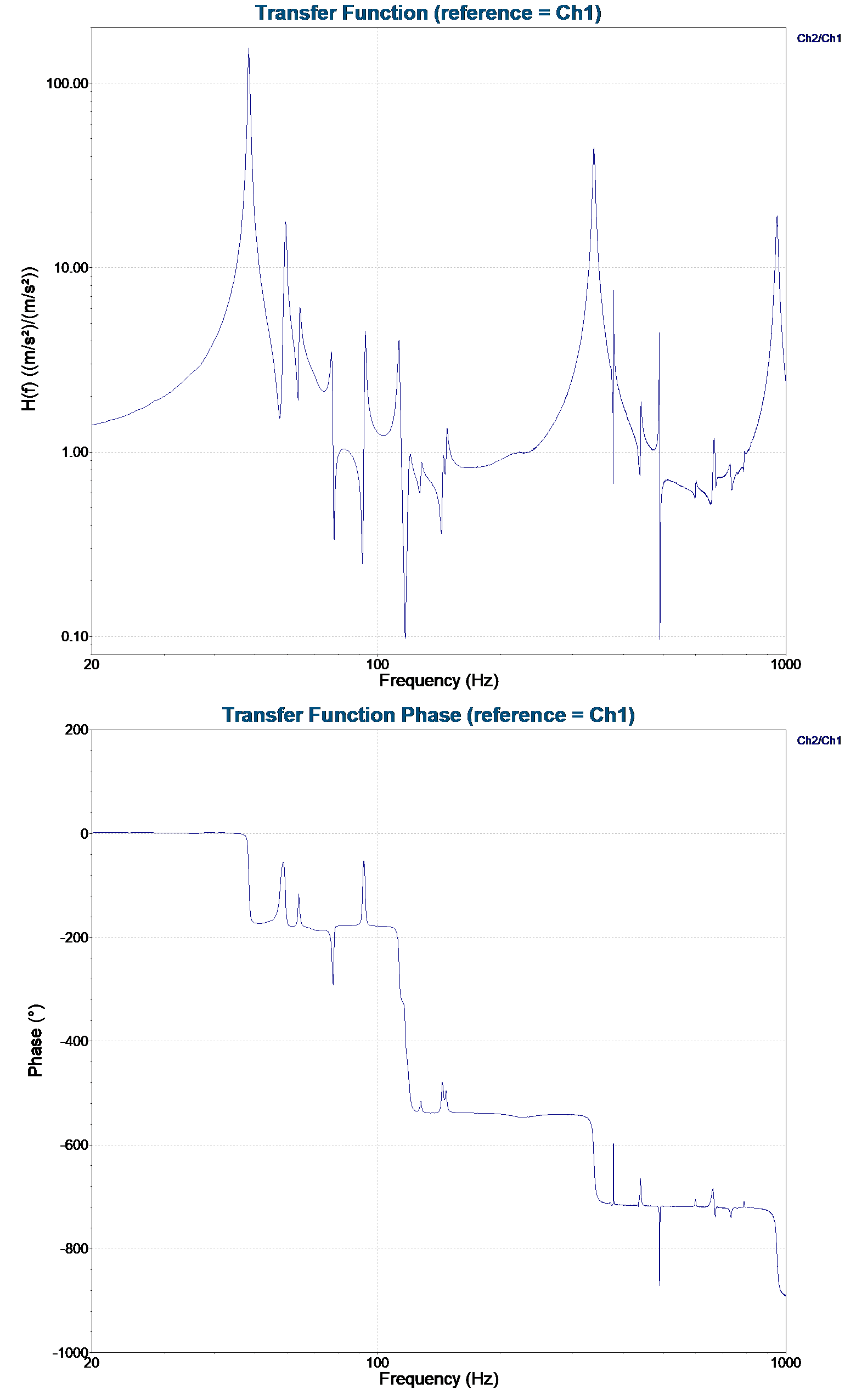# Transfer Function

January 2, 2019

The transfer function uses two graphs to compare (1) the ratio of PSD values for two signals across a specific frequency range and (2) the phase relationships between the two signals.

A transmissibility graph, which is available as a standard VibrationVIEW feature, also shows the PSD relationships between two signals. The phase relationship graph is unique to the transfer function.

### Transfer Function Ratio Values

Test engineers can use a transfer function graph to determine if two different signals are behaving similarly. For example, imagine there are two accelerometers on a shaker head: one at the center and one at the edge. By comparing the signals from the two accelerometers, a test engineer can determine if the shaker head is moving consistently up and down across the shaker head without tilting, rotating, or flexing. The transfer function will also indicate any phase differences between the signals.

If the graph has a value of 1, then the two accelerometers are moving with the same acceleration. Values other than 1 indicate a difference in movement.

If the graph has a value of 0o, then the accelerometers are moving in the same direction. A value of 180o indicates that the accelerometers are moving in opposite directions. Any other value indicates that the accelerometers are moving in some other “off-beat” vibration.

### Transfer Function vs. Transmissibility

To compare the transfer function graph with the transmissibility graph, imagine the following scenario:

A test engineer runs a test to compare the acceleration of a shaker head and device under test (DUT). The transfer function graph has a ratio value of 1 across the spectrum. The transmissibility graph also has a value of 1. From the ratio value, the engineer may conclude that the shaker head and the DUT are vibrating in the same manner. However, they only know that the shaker head and DUT are vibrating with the same acceleration.

With the transfer function phase graph, the engineer can determine if the shaker head and DUT are moving in the same direction at the same time. It is possible for the transfer function to have an acceleration ratio value of 1 and a phase value of 180o. This would indicate opposite directions of motion with the same acceleration.

In Figure 2.1, the phase ratio of the two channels is 0o for the first 80Hz, indicating that the two accelerometers are moving in the same direction. As the aluminum beam approaches a major resonance, the phase ratio changes rapidly. At peak resonance, the phase ratio is 90o. For the remainder of the test, the phase ratio oscillates between 180o and -180o. This indicates that the aluminum beam and the shaker head were vibrating in the same direction for only a short period of time.Figure 2.1. Transmissibility (above) compared to the transfer function (below) for beam vibrations (Ch.2) and shaker head vibrations (Ch. 1). For a majority of the frequency spectrum, the aluminum beam is vibrating out of phase (180o or –180o) with the shaker head.

### A Second Example

The following test results were taken from a run on a vibration test shaker. Channel 1 is the control acceleration and Channel 2 is the response of a cantilever beam. The resulting transfer function and transmissibility graphs display the acceleration ratios of the two accelerometers (Figures 2.2 and 2.3).Figure 2.2. Transmissibility plot of control acceleration (Ch1) and the response of a cantilever beam (Ch2).Figure 2.3. Transfer function plot and phase of control acceleration (Ch1) and the response of a cantilever beam (Ch2).

This transfer function (amplitude) graph is essentially identical to a transmissibility plot because they both compare the acceleration ratios of the two accelerometers. However, the transfer function phase provides more information regarding phase relationships.

### Conclusion

The transfer function graph delivers valuable insights into a variety of test settings. During shaker validation testing, it can help to determine if the vibrations of the shaker head are similar and to troubleshoot for any test or system anomalies. During product testing, the graph not only identifies resonances but also provides deeper insights by determining phase relationships.

The graph has two major advantages over the transmissibility graph. First, the transfer function phase graph provides information about the phase relationship between the two signals. Second, the transfer function acceleration ratio graph is more accurate than the transmissibility graph because it effectively removes the noise factor.

The major disadvantage of the transfer function is the computation time needed to make the necessary calculations for the plots, as they are much more complex than the calculations for the transmissibility graph.